Courses

SRMJEEE Maths Mock Test - 8

35 Questions MCQ Test SRMJEEE Subject Wise & Full Length Mock Tests | SRMJEEE Maths Mock Test - 8

Description
Attempt SRMJEEE Maths Mock Test - 8 | 35 questions in 50 minutes | Mock test for JEE preparation | Free important questions MCQ to study SRMJEEE Subject Wise & Full Length Mock Tests for JEE Exam | Download free PDF with solutions
QUESTION: 1

y = {x(x - 3)}2 increases for all values of x lying in the interval

Solution: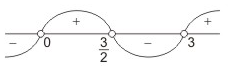Since y = {x(x - 3)}2
∴ dy dx = 2x (x - 3) (2x - 3)
For increasing function dy/dx > 0
or 2x (x - 3) (2x - 3) > 0
or x(x - 3) (x - 3/2) > 0
∴ x∈(0, 3/2) ∪ (3, ∞)

QUESTION: 2

Solution:
QUESTION: 3

If the sum of the coefficients in the expansion of (x+y)n is 1024, then the value of the greatest coefficient in the the expansion is

Solution:
QUESTION: 4

If the equation 3x2 + xy - y2 - 3x + 6y + K = 0 represents pair of lines, then the value of K is

Solution:
QUESTION: 5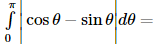Solution:
QUESTION: 6

The equation of a circle with centre (4, 1) and having 3x + 4y -1 = 0 as tangent is

Solution:
QUESTION: 7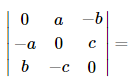Solution:
QUESTION: 8

If |(z-1)/(z-i)| = 1, then the locus of z is

Solution:
QUESTION: 9

The focus of the parabola y2-x-2y+2=0 is

Solution:
QUESTION: 10

If y=a cos (log x) + b sin (log x) where a,b are parametres, then x2y+xy'

Solution:
QUESTION: 11

The derivative of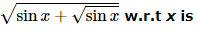Solution:
QUESTION: 12

∫[(1)/(x-x2)]dx=

Solution:
QUESTION: 13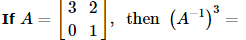Solution:
QUESTION: 14

4tan⁻1(1/5)-tan⁻1(1/239)=

Solution:
QUESTION: 15

What is the value of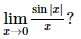Solution:
QUESTION: 16

If f : ℝ → ℝ is defined by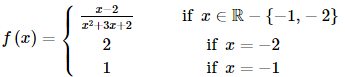then f is continuous on the set

Solution: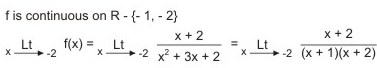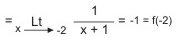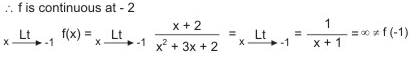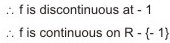QUESTION: 17

If A is a non-singular, then adj A is

Solution:
QUESTION: 18

Let f(x) = x3 + 3x2 + 3x + 2. Then, at x = -1

Solution:

f(x) = (x+1)3 + 1       ∴ f'(x) = 3(x+1)2.
f'(x) = 0    ⇒   x = -1.
Now, f" (-1 - ∈) = 3(-∈)2 > 0, f'(-1 + ∈)2 = 3∈2 > 0.
∴   f(x) has neither a maximum nor a minimum at x = -1.
Let f'(x) = φ ′ (x) = 3(x+1)2     ∴   φ ′ (x) = 6(x+1).
φ ′ (x) = 0    ⇒   x = -1
φ ′ (-1-∈) = 6(-∈) < 0, φ ′ (-1-∈) = 6∈ > 0
∴    φ (x) has a minimum at x = -1

QUESTION: 19

Can a quartile, a decile and a percentile be the median?

Solution:
QUESTION: 20

The gap between the highest and the lowest score is called

Solution:
QUESTION: 21

The number of 4-digit even numbers that can be formed using 0, 1, 2, 3, 4, 5, 6 without repetition is

Solution:

Each even number must have 0, 2, 4 or 6 in is units place
Here total number of digits = 7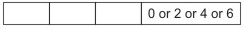When 0 occurs at units place there is no restriction on other places and when 2 or 4 occurs at units place there is restriction on thousands' place as 0 can not be put at thousands' place
Case I When 0 occurs at units place: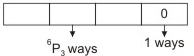∴ The number of numbers formed in this case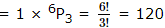Case II. When 0 does not occur at units place: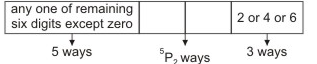The units' place can be filled up by any one of the three digits 2, 4 and 6 in 3 ways
∴ The number of numbers formed in this case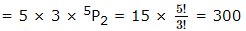∴ The required number = 120 + 300 = 420

QUESTION: 22

The base of triangle is passing through a fixed point (a,b) and its sides are bisected at right angles by lines, y2 - 4xy - 5x2 = 0, then the locus of the vertex of triangle is

Solution: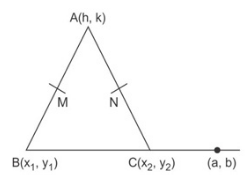y2 - 4xy - 5x2 = 0
⇒ (y - 5x) (y + x) = 0
y - 5x, cuts AB at M at right angle
and y + x = 0 cuts AC at N at right angle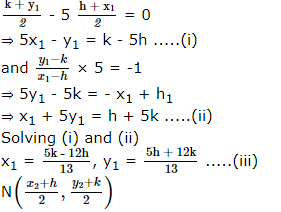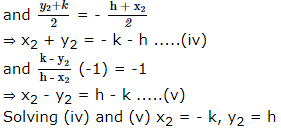By collinearity of (x1, y1), (x2, y2), (a, b) we get h = x, k = y
2x2 + 2y2 + (3a + 2b)x + (2a - 3b)y = 0

QUESTION: 23

If two dice are rolled then the probability that their sum is a prime number is

Solution:
QUESTION: 24

The diameter of the circumcircle of the triangle whose sides 61, 60, 11 is

Solution:
QUESTION: 25

If α , β are the roots of x2 - 2x + 4 = 0 then α5 + β5 =

Solution:
QUESTION: 26

If the roots of the equation x3 - 12x2 + 39x - 28 = 0 are in A.P., then their common difference will be

Solution:
QUESTION: 27

Which one of the following is (A - B) ∪ (B - A)?

Solution:
QUESTION: 28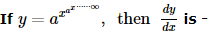Solution:
QUESTION: 29

Lines 2x+y-1=0, ax+3y-3=0 and 3x+2y-2=0 are concurrent

Solution:
QUESTION: 30

The tangent to the curve x=at2, y=2at is perpendicular to x-axis, then its point of contact is

Solution:
QUESTION: 31

A random variable X has the following distribution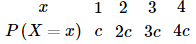The value of c is

Solution:
QUESTION: 32

If tan2θ tanθ=1, the general value of θ is

Solution:
QUESTION: 33

If 4 sinθ=3 cosθ, then sec2θ/4[1-tan2θ] is

Solution:
QUESTION: 34

If a,b,c are the position vectors of vertices of a ∆ABC, the vectorial area of ∆ABC is

Solution:
QUESTION: 35

If the modulus of a and b are equal and angle between them is 120°, and a.b=-8, then the value of |a| is

Solution:Use Code STAYHOME200 and get INR 200 additional OFF Use Coupon Code

Track your progress, build streaks, highlight & save important lessons and more!

Similar ContentRelated tests